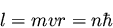Next: Wave-Particle Duality Up: The Motivation for Quantum Previous: The Photoelectric Effect

## Quantization of Electronic Angular Momentum

Rutherford proposed that electrons orbit about the nucleus of an atom. One problem with this model is that, classically, orbiting electrons experience a centripetal acceleration, and accelerating charges lose energy by radiating; a stable electronic orbit is classically forbidden. Bohr nevertheless assumed stable electronic orbits with the electronic angular momentum quantized as(2)

Quantization of angular momentum means that the radius of the orbit and the energy will be quantized as well. Bohr assumed that the discrete lines seen in the spectrum of the hydrogen atom were due to transitions of an electron from one allowed orbit/energy to another. He further assumed that the energy for a transition is acquired or released in the form of a photon as proposed by Einstein, so that(3)

This is known as the Bohr frequency condition. This condition, along with Bohr's expression for the allowed energy levels, gives a good match to the observed hydrogen atom spectrum. However, it works only for atoms with one electron.Next: Wave-Particle Duality Up: The Motivation for Quantum Previous: The Photoelectric Effect